The solution to a matrix equation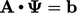by descent methods involves ﬁnding the minimum of the equation in quadratic form. This can be illustrated using the contours of the paraboloid describing the quadratic for the case with values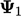and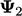.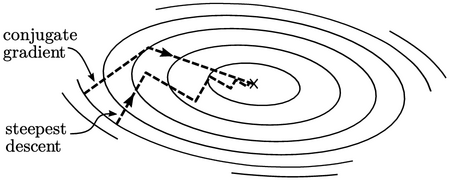The search for the minimum involves a series of updates to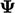of the form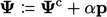(5.32)
whereis the next update using latest (current) values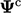. The column vector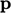provides the direction of the line search towards the minimum; the scalar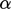provides the magnitude of the line is that direction.

### Steepest descent

The intuitive way to reach the minimum is to follow the direction of steepest descent. The method is fairly simple because the direction is deﬁned by the negative of gradient of the quadratic form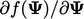which is the residual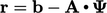.

The distance to “walk” is naturally until the lowest point is reached, corresponding to5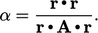(5.33)
The method reaches the minimum point along a zigzag path where consecutive directions are orthogonal. The directions do not change and result in a larger number of very short steps, the more the paraboloid is stretched in one direction.

### The conjugate direction

The conjugate gradient (CG) method chooses search directions that are conjugate with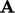. This means each new directioncorresponds to the previous one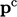, satisfying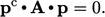(5.34)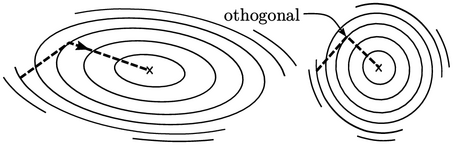This can be imagined as the directions being orthogonal with the stretch in the paraboloid removed. For 2 values, CG ﬁnds the minimum in 2 steps, rather than several zigzag steps.

CG provides the basis for practical matrix solvers for CFD, described in Sec. 5.16 . For a detailed explanation of the CG method, see the reference below.

5Jonathan Richard Shewchuk An introduction to the conjugate gradient method without the agonizing pain, 1994.

Notes on CFD: General Principles - 5.15 Conjugate gradient method